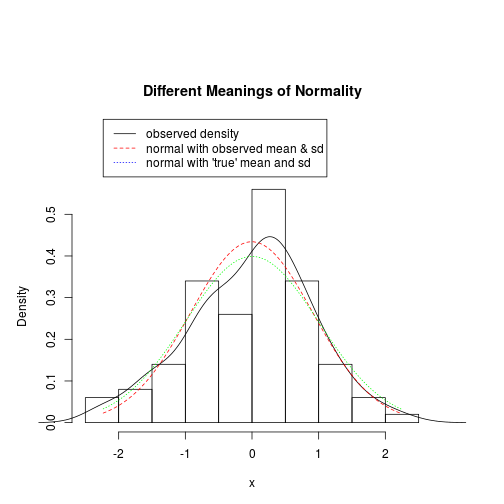## Title: plot-histogramWithLinesAndLegend.R
## Author: Paul E Johnson <pauljohn at ku.edu>
## Date: 2013-02-11
## Description: Show ways to customize a histogram.
##   This one runs into problem that there is not enough
##   room in the top of the figure for a legend. We have
##   choices to make margins bigger or to allow the
##   plotter to draw outside the "plot region" (xpd=TRUE)

## Thanks to r-help members for tips.

x <- rnorm(100)
###Allows writing outside plotting region

par(mai=c(1,1,2.5,1)) ## margins
par(xpd=TRUE) ## write outside plot region

myhist <- hist(x, prob = TRUE, main="Different Meanings of Normality")

lines(density(x))

xseq1 <- seq( min(x), max(x), length.out=100)

m1 <- mean(x)

sd1 <- sd(x)

obsNormal <- dnorm(xseq1, mean=m1, sd=sd1)

lines( xseq1, obsNormal, lty=2, col="red")

truNormal <- dnorm(xseq1)

lines(xseq1, truNormal, lty=3, col="green")

legend(min(xseq1),1.3*max(myhist\$density), legend=c("observed density", "normal with observed mean & sd", "normal with 'true' mean and sd"), lty=c(1,2,3), col=c("black", "red", "blue"))